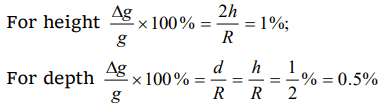## Gravitation Questions and Answers Part-10

1. Acceleration due to gravity on moon is 1/6 of the acceleration due to gravity on earth. If the ratio of densities of earth $\left(\rho_{e}\right)$ and moon $\left(\rho_{m}\right)$ is $\left(\frac{\rho_{e}}{\rho_{m}}\right)=\frac{5}{3}$  then radius of moon Rm in terms of Re will be
a) $\frac{5}{18}R_{e}$
b) $\frac{1}{6}R_{e}$
c) $\frac{3}{18}R_{e}$
d) $\frac{1}{2\sqrt{3}}R_{e}$

Explanation:2. The acceleration of a body due to the attraction of the earth (radius R) at a distance 2 R from the surface of the earth is (g = acceleration due to gravity at the surface of the earth)
a) $\frac{g}{9}$
b) $\frac{g}{3}$
c) $\frac{g}{4}$
d) g

Explanation:3. The depth at which the effective value of acceleration due to gravity is $\frac{g}{4}$ is
a) R
b) $\frac{3R}{4}$
c) $\frac{R}{2}$
d) $\frac{R}{4}$

Explanation:4. Weight of a body of mass m decreases by 1% when it is raised to height h above the earth’s surface. If the body is taken to a depth h in a mine, change in its weight is
a) 2% decrease
b) 0.5% decrease
c) 1% increase
d) 0.5% increase

Explanation:5. If both the mass and the radius of the earth decrease by 1%, the value of the acceleration due to gravity will
a) Decrease by 1%
b) Increase by 1%
c) Increase by 2%
d) Remain unchanged

Explanation:6. The density of a newly discovered planet is twice that of earth. The acceleration due to gravity at the surface of the planet is equal to that at the surface of the earth. If the radius of the earth is R, the radius of the planet would be
a) 2 R
b) 4 R
c) $\frac{1}{4}R$
d) $\frac{1}{2}R$

Explanation:7. Two planets of radii in the ratio 2 : 3 are made from the material of density in the ratio 3 : 2. Then the ratio of acceleration due to gravity $g_{1}\diagup g_{2}$  at the surface of the two planets will be
a) 1
b) 2.25
c) 4/9
d) 0.12

Explanation:8. A person will get more quantity of matter in kg – wt. at
a) Poles
b) At latitude of $60^{\circ}$
c) Equator
d) Satellite

Explanation: Because the body weighs zero in satellite

9.At what depth below the surface of the earth, acceleration due to gravity g will be half its value 1600 km above the surface of the earth
a) $4.2\times 10^{6}m$
b) $3.19\times 10^{6}m$
c) $1.59\times 10^{6}m$
d) None of thesea) $\frac{1}{800}rad / s$
b) $\frac{1}{600}rad / s$
c) $\frac{1}{400}rad / s$
d) $\frac{1}{100}rad / s$